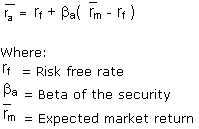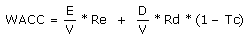# Answer to Question #6207 in Economics of Enterprise for LaMarcus Streeter

Question #6207
Analyze the various ways to determine the cost of capital and determine which is the most difficult to get right. Explain your rationale.
1
2012-02-07T14:19:36-0500
The cost of capital determines how a company can raise money (through a stock issue, borrowing, or a mix of the two). This is the
rate of return that a firm would receive if it invested in a different vehicle
with similar risk. Cost of capital = interest rate at zero level risk + premium for business risk + premium for
financial risk. There are other different ways to determine the cost of capital:

1.The cost of debt is relatively simple to calculate, as it is composed of the rate of interest paid. It is computed by
taking the rate on a risk free bond whose duration matches the term structure of
the corporate debt, then adding a default premium. The formula can be written as
(Rf + credit risk rate)(1-T), where T is the corporate tax rate and Rf is the
risk free rate.

2.The cost of equity is more challenging to calculate as equity does not pay a set return to its investors. It is therefore inferred by comparing the investment to other investments
(comparable) with similar risk profiles to determine the &quot;market&quot; cost of
equity. It is commonly equated using the Capital Asset Pricing Model (CAPM) formula:An alternative to the estimation of the required return by the CAPM as above, is the use of the Fama&ndash;French three-factor
model.
r = Rf + &beta; (Km - Rf ) + bs * SMB + bv * HML + &alpha;.
Here r is the portfolio&#039;s rate of return, Rf is the risk-free return rate, and Km is the return of the whole stock market. The &quot;three factor&quot; &beta; is analogous to the classical &beta; but not equal to it, since there are now two additional factors to do some of the work. SMB stands for &quot;small (market capitalization) minus big&quot; and HML for &quot;high (book-to-market ratio) minus low&quot;; they measure the historic excess returns of small caps over
big caps and of value stocks over growth stocks.

3. The Weighted Average Cost of Capital (WACC) is used in finance to measure a firm&#039;s cost of capital.
Its calculation is an iterative procedure which requires estimation of the fair
market value of equity capital:Where:
Re = cost of equity
Rd = cost of debt
E = market value of the firm&#039;s equity
D = market value of the firm&#039;s debt
V = E + D
E/V = percentage of financing that is equity
D/V = percentage of financing that is debt
Tc = corporate tax rate

Need a fast expert's response?

Submit order

and get a quick answer at the best price

for any assignment or question with DETAILED EXPLANATIONS!